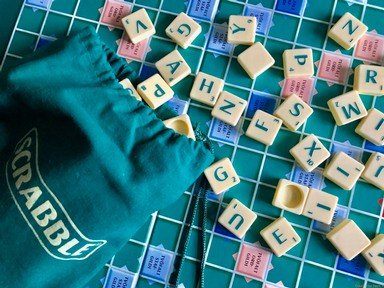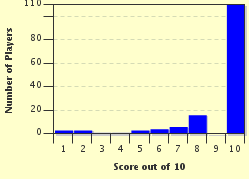FREE! Click here to Join FunTrivia. Thousands of games, quizzes, and lots more!# The Value of a Word Trivia Quiz

### How well do you know the point values associated with each letter tile in Scrabble? Can you correctly match the word on the left with the word on the right that is worth the same number of points? (with no multipliers used)

A matching quiz by reedy. Estimated time: 4 mins.

Author
reedy
Time
4 mins
Type
Match Quiz
Quiz #
387,815
Updated
Dec 03 21
# Qns
10
Difficulty
Average
Avg Score
8 / 10
Plays
1222
Last 3 plays: nasty_liar (8/10), Chucklemel (7/10), Guest 174 (10/10).
Mobile instructions: Press on an answer on the right. Then, press on the gray box it matches on the left.
(a) Drag-and-drop from the right to the left, or (b) click on a right side answer box and then on a left side box to move it.
 Questions Choices 1. PEOPLE VALUE 2. WHO CORRECT 3. ENJOY JACKPOT 4. PLAYING ACCEPT 5. GAMES POINT 6. MIGHT RIGHT 7. GET EXPERT 8. THIS CAPABLE 9. MATCH CONCUR 10. QUIZ TRUE

Quiz Answer Key and Fun Facts
1. PEOPLE

PEOPLE = 3+1+1+3+1+1 for a total of 10 points.

CONCUR = 3+1+1+3+1+1 which also equals 10 points.
2. WHO

WHO = 4+4+1 for a total of 9 points.

RIGHT = 1+1+2+4+1 which also equals 9 points.
3. ENJOY

ENJOY = 1+1+8+1+4 for a total of 15 points.

EXPERT = 1+8+3+1+1+1 which also equals 15 points.
4. PLAYING

PLAYING = 3+1+1+4+1+1+2 for a total of 13 points.

CAPABLE = 3+1+3+1+3+1+1 which also equals 13 points.
5. GAMES

GAMES = 2+1+3+1+1 for a total of 8 points.

VALUE = 4+1+1+1+1 which also equals 8 points.
6. MIGHT

MIGHT = 3+1+2+4+1 for a total of 11 points.

CORRECT = 3+1+1+1+1+3+1 which also equals 11 points.
7. GET

GET = 2+1+1 for a total of 4 points.

TRUE = 1+1+1+1 which also equals 4 points.
8. THIS

THIS = 1+4+1+1 for a total of 7 points.

POINT = 3+1+1+1+1 which also equals 7 points.
9. MATCH

MATCH = 3+1+1+3+4 for a total of 12 points.

ACCEPT = 1+3+3+1+3+1 which also equals 12 points.
10. QUIZ

QUIZ = 10+1+1+10 for a total of 22 points.

JACKPOT = 8+1+3+5+3+1+1 which also equals 22 points.
Source: Author reedy

This quiz was reviewed by FunTrivia editor WesleyCrusher before going online.
Any errors found in FunTrivia content are routinely corrected through our feedback system.
Most Recent Scores
Jun 07 2023 : nasty_liar: 8/10
May 12 2023 : Chucklemel: 7/10
May 11 2023 : Guest 174: 10/10
Apr 14 2023 : calmdecember: 8/10
Apr 14 2023 : hickorystick: 5/10

Score DistributionRelated Quizzes
This quiz is part of series Many Magnificent Matches, Part 5:

My fifth installment of ten match quizzes - enjoy!

1. The Value of a Word Average